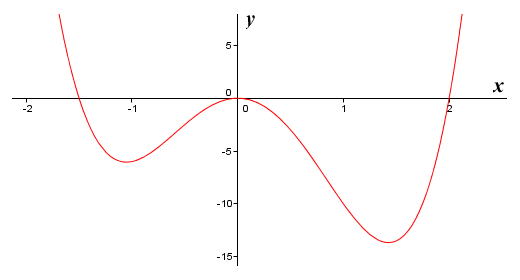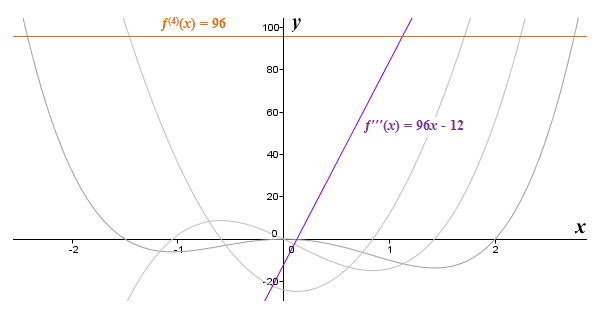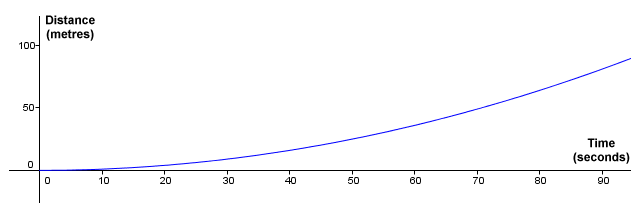# Higher Derivatives

When we take the derivative of a function, we end up with another function. We will call this new function the first derivative, for reasons that will hopefully become clear in due course. You should by now be comfortable with the idea that the derivative of a function is another function that will give us the slope of the original function for any value of x for which the original function is defined. Since the first derivative is itself a function, it stands to reason that we should be able to plot the graph of this function. It also seems reasonable to assume that we should be able to take its derivative. Since this derivative would be a derivative of a derivative, we call it the second derivative of the original function. Let's look at an example. Here is the graph of the function ƒ(x) = 4x 4 - 2x 3 - 12x 2:The graph of the function ƒ(x) = 4x 4 - 2x 3 - 12x 2

If we apply the basic rules of differentiation to this function to get the derivative, we get:

ƒ′(x)  =  16x 3 - 6x 2 - 24x

The notation we have used to denote the first derivative of a function is the Lagrange notation mentioned elsewhere in these pages. In this notation, a single prime symbol ("ʹ") is placed immediately after the function symbol to indicate that this is the first derivative of the original function. To indicate the second derivative, we place two prime symbols immediately after the function symbol. To indicate the third derivative, three prime symbols are used. We also have the option of using Liebniz's notation, and we will see what that looks like in due course. For now, we will stick with the Lagrange notation.

Here are the graphs of the original function and its first derivative:The graphs of the function ƒ(x) = 4x 4 - 2x 3 - 12x 2 and its first derivative

We will apply the basic rules of differentiation again in order to find the derivative of the function y = 16x 3 - 6x 2 - 24x. This will give us the second derivative of the original function, which we can write as:

ƒ′′(x)  =  48x 2 - 12x - 24

Here are the graphs of the original function and its first and second derivatives:The graphs of the function ƒ(x) = 4x 4 - 2x 3 - 12x 2 and its first and second derivatives

We can also find the derivative of the derivative of the derivative of a function. We call this derivative the third derivative. We find the third derivative by taking the derivative of y = 48x 2 - 12x - 24 (the second derivative), which gives us:

ƒ′′′(x)  =  96x - 12

We can even take the derivative of the third derivative (the fourth derivative), which is:

ƒ (4)(x)  =  96

The third derivative produces a linear graph. The fourth derivative is a constant value, so the resulting graph is also linear, but this time it is parallel to the x axis. Note that from the fourth derivative onwards, we denote the ordinal using a superscripted number enclosed within parentheses. We do this for the sake of clarity - it is simply easier to read a number than a long string of prime symbols. The use of parentheses ensures that the number is not mistaken for an exponent. Although we are not usually all that interested in derivatives that produce linear graphs, we present the graphs of the third and fourth derivatives below for the sake of completeness. Note that the fifth derivative, being the derivative of a constant, will be zero.The third and fourth derivatives of the function ƒ(x) = 4x 4 - 2x 3 - 12x 2 are linear functions

Any derivatives beyond the first derivative of a function are referred to as higher derivatives or higher order derivatives. You might well be wondering, quite reasonably, why we would be interested in the derivative of a derivative, or the derivative of a derivative of a derivative. We know, of course, that the first derivative of a function tells us how fast the function is changing - i.e. the slope of the function - for any value of x for which the function is defined. In other words, it tells us the function's instantaneous rate of change. Likewise, the second derivative tells us how fast the first derivative of the function (which is a function itself, remember) is changing. It is the rate of change of the rate of change of the original function. The third derivative tells us how fast the second derivative of the function is changing. It is the rate of change of the rate of change of the rate of change of the original function.

The higher the order of the derivative, the more difficult it becomes to understand what the derivative actually represents. Fortunately, we rarely have to deal with anything higher than the third derivative. We know already that the first derivative gives us the rate of change of a function, but the second and third derivatives can be quite useful too. We can use the second derivative, for example, to help us to identify potential maxima and minima of a function. But that's something that we'll discuss elsewhere. Higher order derivatives also find practical applications in areas such as physics. The classic example is that of speed (or velocity) versus acceleration. Think about some object moving at a constant speed of zero-point-two metres per second (0.2 m/s) in a straight line. If we plot a displacement-time graph (i.e. a graph of the distance travelled against the time elapsed), it will be linear, as we can see below.Displacement-time graph for object travelling at a constant speed of 0.2 m/s

You can probably think of lots of examples of situations in which objects are travelling at some (more or less) constant velocity. A car travelling on the motorway can maintain a constant speed for a considerable time, if there is not too much traffic. Commercial passenger aircraft on long flights routinely cruise at a constant speed for several hours, once they have achieved the required altitude. But how does a stationary object acquire a constant speed? It must undergo acceleration. Unless you have been living in a remote wilderness all your life, you have probably seen a train leaving a station, or a car pulling away from traffic lights. At first, the vehicle moves slowly. Then it gradually picks up speed (accelerates) until the required speed is achieved.

Supposing we once again plot a displacement-time graph, this time for a train leaving a station. We will assume that the train undergoes a constant acceleration of two metres per second per second (0.2 m/s 2), which gives us the following displacement function:

 ƒ(x)  =  0.02 x 2 2

Here is the displacement-time graph:Displacement-time graph for object with a constant acceleration of 0.2 m/s 2

If we rewrite the function to get rid of the fraction, we get:

ƒ(x)  =  0.01x2

Now, since velocity is the rate of change of displacement, the first derivative of the displacement function should give us the velocity function. If we differentiate ƒ(x) = 0.01x 2 we get:

ƒ′(x)  =  0.02x

And, since acceleration is the rate of change of velocity, the derivative of the velocity function should give us the acceleration function. If we differentiate ƒ′(x) = 0.02x we get:

ƒ′(ƒ′(x))  =  0.02

However, since we know that the derivative of the derivative of a function is the second derivative of the function, we can write this more elegantly as:

ƒ′′(x)  =  0.02

This result, of course, gives us the acceleration. So, we can clearly see that the second derivative of the displacement-time function for a body moving with uniform acceleration will give us the actual rate of acceleration.

You might have noticed that the functions we have looked at so far have all been polynomial functions. We saw that differentiating the function ƒ(x) = 4x 4 - 2x 3 - 12x 2, which is a fourth degree polynomial function, gave us the first derivative function ƒ′(x) = 16x 3 - 6x 2 - 24x, which is a third degree polynomial. The second derivative function ƒ′′(x) = 48x 2 - 12x - 24 is a second degree polynomial function, and the third derivative ƒ′′′(x) = 96x - 12 is a first degree (i.e. linear) polynomial function. Differentiating a polynomial function of degree n always results in a polynomial function of degree n - 1 unless n is zero, in which case the function is a constant, and the derivative will be zero. Since zero is also a constant, there is no point in trying to find any higher order derivatives.

Not all functions play so nicely. Consider the following rational function:

 ƒ(x)  = x 2 - 7 x + 3

Taking the first derivative, we get:

 ƒ′(x)  = 2x - x 2 - 7 x + 3 (x + 3) 2

Taking the second derivative, we get:

 ƒ′′(x)  = 2 - 4x + 2 · (x 2 - 7) x + 3 (x + 3) 2 (x + 3) 3

So, as you can see, rather than getting simpler, the higher derivatives of a rational function can become increasingly complex. The trigonometric sine and cosine functions, on the other hand, exhibit very different behaviour. In each case, the sequence of derivatives, like the function itself, is cyclical. To illustrate this point, we present here the sine function and its first four derivatives:

ƒ(x)  =  sin (x)

ƒ′(x)  =  cos (x)

ƒ′′(x)  =  -sin (x)

ƒ′′′(x)  =  -cos (x)

ƒ (4)(x)  =  sin (x)

From the fourth derivative onwards, the entire cycle simply repeats itself as the ordinal increases. You can probably see that the cosine function will behave in exactly the same way. The sequence will be exactly the same. The only difference is that this time it will start with cos (x). Here is the cosine function and its first four derivatives.

ƒ(x)  =  cos (x)

ƒ′(x)  =  -sin (x)

ƒ′′(x)  =  -cos (x)

ƒ′′′(x)  =  sin (x)

ƒ (4)(x)  =  cos (x)

Before we leave this topic, we should perhaps say a bit more about notation. We mentioned earlier that we can use Liebniz's notation to denote the higher derivatives rather than the Lagrange notation we have used up to now. Supposing we have some function, ƒ(x). As we have seen elsewhere in these pages, we can express the first derivative of ƒ(x) as follows:

 dy = d (ƒ(x)) dx dx

We can derive an expression for the second derivative of ƒ(x) as follows:

 ( dy )′ = d ( dy ) = d 2 y (ƒ(x)) dx dx dx dx 2

Following on from this, the third derivative of ƒ(x) would be written as:

 d 3 y (ƒ(x)) dx 3

The fourth derivative of ƒ(x) would likewise be:

 d 4 y (ƒ(x)) dx 4

Hopefully you can see the pattern that is emerging here, so we won't labour the point by extending the list any further. Generally speaking, the form of notation to be used is often a matter of personal choice. There may be situations, however, in which one form or the other is more appropriate, or simply more convenient. Naturally, if you are asked by your tutor to use a specific form of notation, then you should do so.